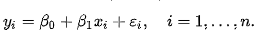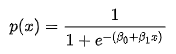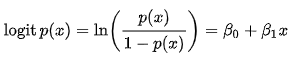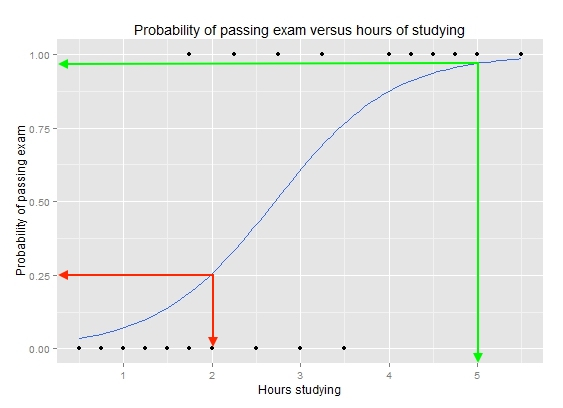# Logistic Regressor

### Understanding the Logistic Regressor

It is easier to confuse Logistic Regression as a Regression algorithm due to the “Regression” attached to its name, but as mentioned earlier, it is a pure classification model that can predict dependent variables that falls into specific categories.

To put it in a much simpler way, Logistic regression works when your dependent variable(s) is/are categories like yes or no, male or female or variables that fall into multiple categories like States, Currencies, Brands or Countries etc.
The Logistic Regression Equation:

The logistic regression equation is derived from the Linear regression and Sigmoid function.

The Linear equation gives us :Sigmoid function after substituting for y from the above equation :The resulting solution is called the Logistic Regression EquationNow let us try to understand the equation with one of the simplest illustrations available on the internet. The following graph depicts the plot between the time a student spends studying versus whether he/she passes or fails the test.

Clearly what we are trying to predict falls in a binary category since there are only two possible outcomes either the student fails(0) or passes(1).The logistic regression equation represented by the blue line in the graph determines the category by pointing towards the probability. Consider from the given graph, if a student studies for only 2 hours the probability for passing the test is only 0.25 or 25 %, represented by the red lines. If the student studies for 5 hours the probability of passing the test is above 0.9 or 90%. Thus probability determines whether the student passes or fails. For predictive analysis, we fix a critical value or threshold probability score, say for example 50% or 0.5. If the probability falls above 0.5 the student passes otherwise the student fails.